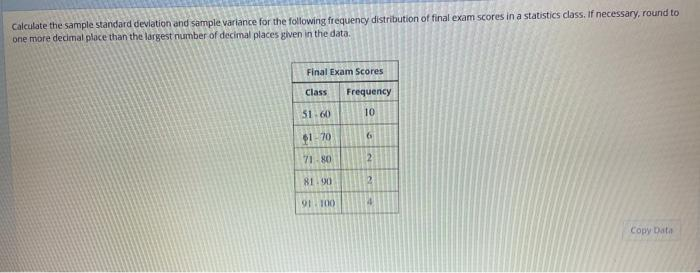# Question Solved1 Answercalculate the sample standard deviation and sample variance for the following frequency distribution of final exam scores in a statistics class if necessary around one more decimal place than the largest number of decimal places given in the data? Calculate the sample standard deviation and sample variance for the following frequency distribution of final exam scores in a statistics class. If necessary, round to one more decimal place than the largest number of decimal places given in the data Final Exam Scores Class Frequency 10 51.60 Es 61-70 7180 2 81.00 2 91100 Copy DataCQXS9D The Asker · Probability and Statistics
calculate the sample standard deviation and sample variance for the following frequency distribution of final exam scores in a statistics class if necessary around one more decimal place than the largest number of decimal places given in the data?Transcribed Image Text: Calculate the sample standard deviation and sample variance for the following frequency distribution of final exam scores in a statistics class. If necessary, round to one more decimal place than the largest number of decimal places given in the data Final Exam Scores Class Frequency 10 51.60 Es 61-70 7180 2 81.00 2 91100 Copy Data
More
Transcribed Image Text: Calculate the sample standard deviation and sample variance for the following frequency distribution of final exam scores in a statistics class. If necessary, round to one more decimal place than the largest number of decimal places given in the data Final Exam Scores Class Frequency 10 51.60 Es 61-70 7180 2 81.00 2 91100 Copy Data